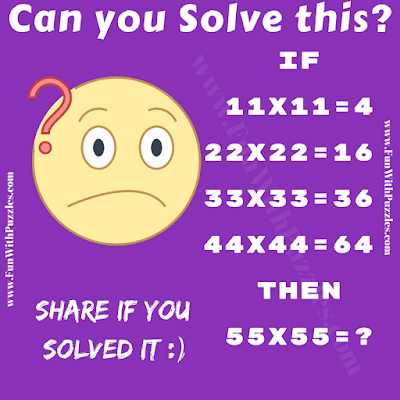This is a very interesting logic of Mathematics Riddle. In this logical Mathematical Riddle in which you are presented with Maths equations which are logically correct. These Maths Equations have some logical pattern. Your challenge is to decode the logical pattern and then calculate the value of the last equation. Can you solve this Logic of Mathematics Riddle?Can you solve this logic of Mathematics Riddle?

The answer to this "Logic of Mathematics Riddle for Teens", can be viewed by clicking on the button. Please do give your best try before looking at the answer.